# Functions and Their Graphs

In mathematics, a function is a particular type of relation with some rules. For example, mathematically, a function f is a relation from a non-empty set A to a non-empty set B such that the domain of f is A and no two distinct ordered pairs in f have the same first element. Also, we have different types of functions that can be defined based on their properties. In this article, you will learn about the graphs of different types of functions.

## List of Functions and Their Graphs

Some of the important functions are listed below:

• Identity function
• Constant function
• Polynomial function
• Rational functions
• Modulus function
• Signum function
• Greatest integer function

Let’s have a look at the meaning and graphs of these functions.

### Identity Function

Suppose the real valued function f : R → R by y = f(x) = x for each x ∈ R (i.e. the set of real numbers). Such a function is called the identity function. Also, the domain and range of this function f are R. The graph of this type of function is a straight line passing through the origin as shown in the below figure.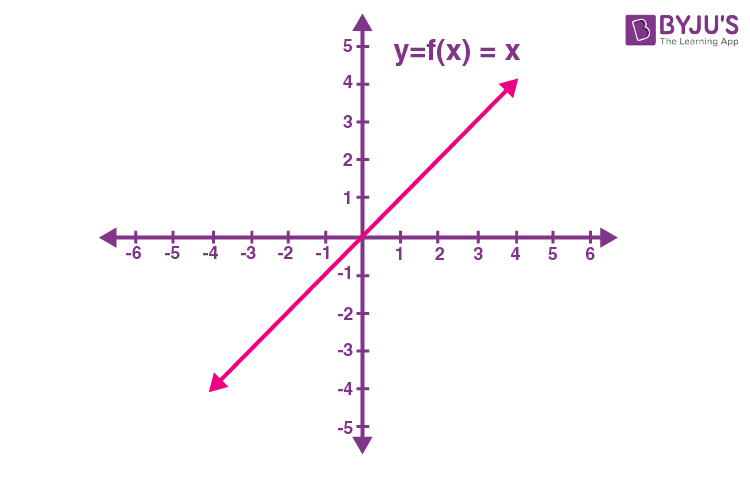### Constant Function

The function f: R → R by y = f (x) = c, x ∈ R where c is a constant and each x ∈ R is called a constant function. The domain of this function is R and its range is {c}. However, the graph of a constant function y = f(x) = 2 is given below.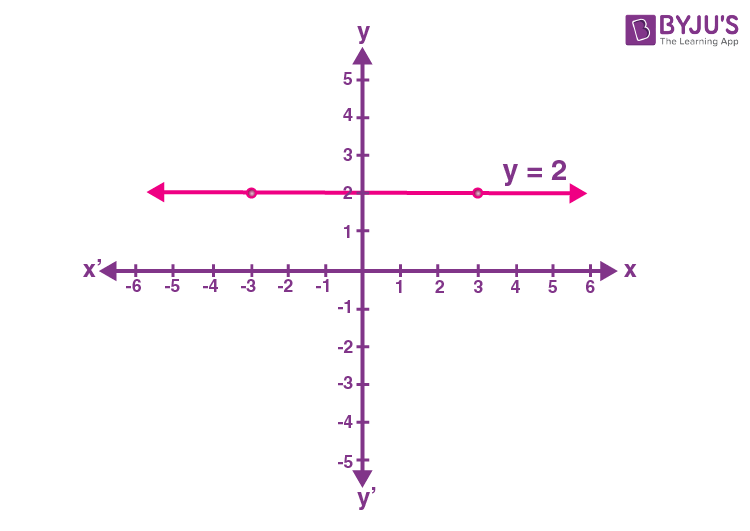### Polynomial Function

A function f : R → R is said to be polynomial function if for each x in R, y = f(x) = a0 + a1x + a2x2 + …+ anxn, where n is a non-negative integer and a0, a1, a2,…,an ∈ R. The graph of this type of function is a parabola. The graph of a certain polynomial function with degree 2 is given below: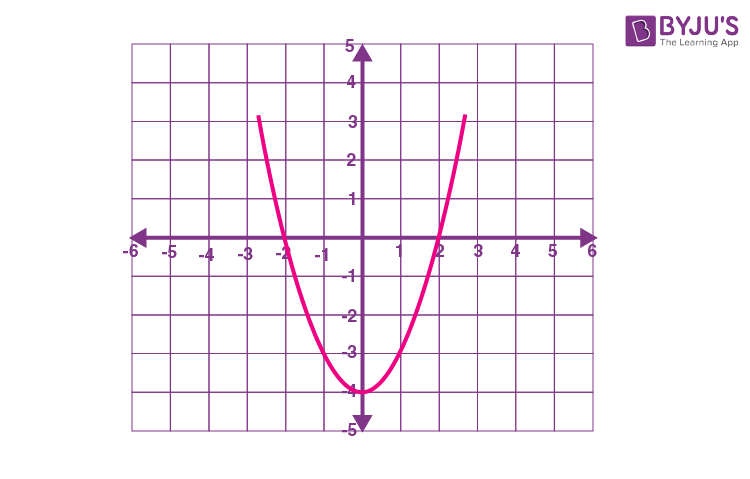### Rational Functions

A function is of the form f(x)/g(x), where f(x) and g(x) are polynomial functions of x defined in a domain such that g(x) ≠ 0 is called a rational function. The example graph of a rational function is given below: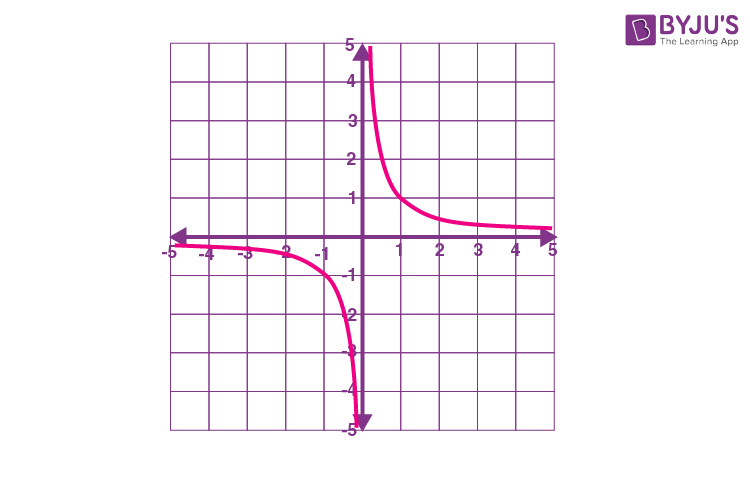### Modulus Function

The function f: R → R defined by y = f(x) = |x| for each x ∈ R is called the modulus function. The graph of a modulus function y = |x| is given below.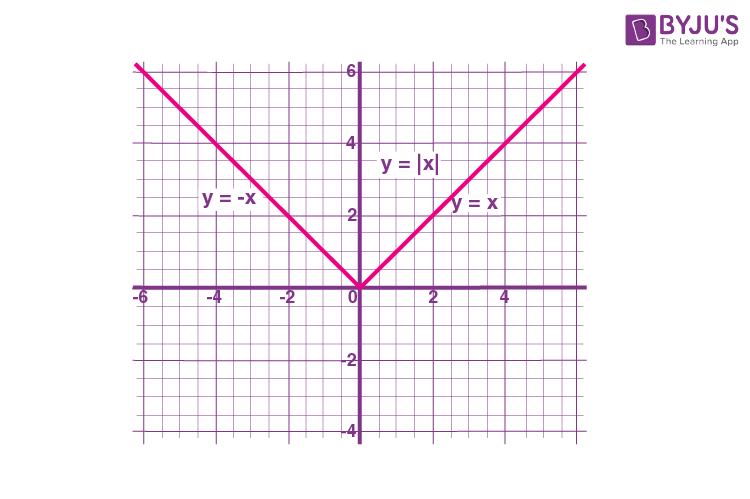Get the complete information about modulus function here.

### Signum Function

The function f: R → R defined by

$f(x)=\left\{\begin{matrix} -1; &x < 0 \\ 0& x=0\\ 1; & x>0 \end{matrix}\right.$

is called the signum function. The domain of this function is R and the range is the set {–1, 0, 1}. The figure given below shows the graph of the signum function.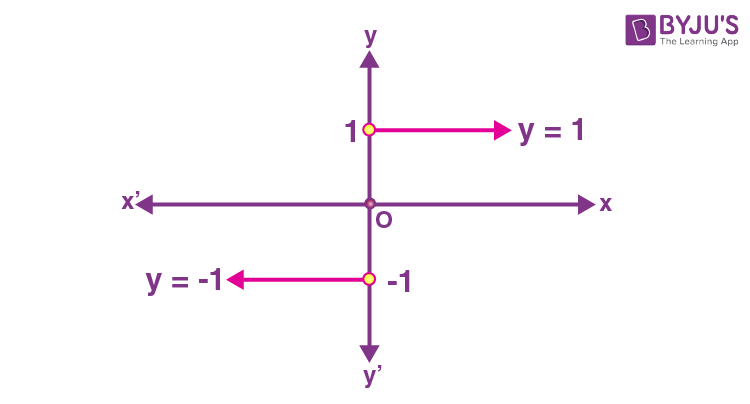### Greatest Integer Function

The function f: R → R defined by f(x) = [x], x ∈R assumes the greatest integer value, less than or equal to x. Such a function is called the greatest integer function. Below is the graph for some greatest integer functions.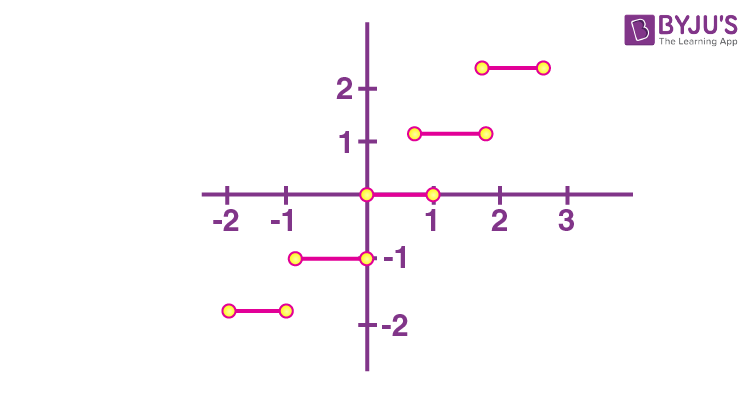Also, check: Greatest integer function

Similarly, we can draw the graphs for other types of functions such as cubic function, even and odd functions, periodic functions, etc.